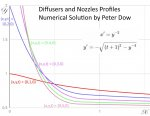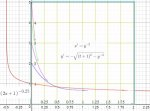# Mathematics of profiles of water diffusers and nozzles.

#### Peter Dow Aberdeen

##### New member
I derived this system of differential equations this week as I was researching possible profiles for water diffusers & nozzles, used when joining pipes with different bores.

Mathematics: Solve this system of differential equations.

$$\displaystyle x' = y^{-2}$$

$$\displaystyle y' = - \sqrt {(t+1)^2- y^{-4}}$$

$$\displaystyle x'$$ and $$\displaystyle y'$$ are derivatives with respect to $$\displaystyle t$$.​

I have obtained a numerical solution (which was non-trivial because of the numerical instability of the Euler method with this system of differential equations) but I am curious to know "does an analytical solution exist?",which would be more efficient and convenient to use.

Derivation of the system of differential equations

Water is accelerated in a nozzle or a pipe of reducing width, which is rotationally symmetrical about the X-axis, with the bore, the inner diameter and the inner radius proportional to a function $$\displaystyle y(x)$$ .

Neglecting viscosity and considering averages for simplicity, the velocity of the water in the X-axis is inversely proportional to the bore's area of cross section and to $$\displaystyle y^2$$.

$$\displaystyle x' = y^{-2}$$

The average velocity r', of a radial element, a thin slice of water "pie", is composed of the vector addition of the velocity along the X-axis x' and in the radial direction y', which are related in magnitude by Pythagoras,

$$\displaystyle r'^2 = x'^2 + y'^2$$

So

$$\displaystyle y' = \sqrt{r'^2 - x'^2}$$

Assume an acceleration $$\displaystyle r' = at + u$$, at a time t, with acceleration a and initial velocity u, but for simplicity here, both a and u are assumed to be 1.

$$\displaystyle r' = t + 1$$

Therefore

$$\displaystyle y' = - \sqrt{ (t+1)^2 - y^{-4}}$$

choosing the negative root corresponding to an radially inward y' when water accelerates in a nozzle.

Numerical SolutionThe numerical instability was managed by writing a computer program which could calculate in t-increments corresponding to the square root of linear increments in $$\displaystyle t^2$$.

$$\displaystyle t_{i+1} = t_i + \Delta t_i$$ where $$\displaystyle \Delta t_i = \min(h_1,\sqrt{t_i^2+h_2}-t_i)$$ and $$\displaystyle h_1$$, $$\displaystyle h_2$$ are step size constants.

Approximate solution for $$\displaystyle (x,y,t) = (0,1,0)$$

With the initial conditions $$\displaystyle t=0, x=0, y=1$$ then $$\displaystyle x' = r' = 1$$ and $$\displaystyle y'=0$$.

Assuming that then $$\displaystyle y' << x'$$ for all $$\displaystyle t>0$$ then approximately

$$\displaystyle x' = t + 1$$

Integrating with respect to t and substituting for t (or simplifying this equation for linear acceleration, $$\displaystyle x'^2 - u^2 = 2ax$$ with $$\displaystyle a=1$$ and $$\displaystyle u=1$$ ) gives

$$\displaystyle x'^2 - 1 = 2x$$

Substituting for $$\displaystyle x' = \sqrt{2x+1}$$ in the system equation $$\displaystyle x' = y^{-2}$$ and rearranging for y gives

$$\displaystyle y = (2x+1)^{-0.25}$$

As this graph shows, this is a good approximate solution for these starting conditions.Note on numerical instability
The graph also shows how numerical stability interrupted the numerical solution part plotted using the free on-line Two Dimensional Differential Equation Solver and Grapher V 1.0. Investigate the numerical instability by selecting the option "System of first order DEs: x' = f(x, y, t), y' = g(x, y, t)" and typing for x', y' -
x' = y^(-2)
y' = -1*sqrt((t+1)^2-y^(-4))
with initial values (x=0, y $$\displaystyle \ge$$1)

#### CurveGuy

##### New member
Hmm, an analytical solution does not stand out in my old mind. But, take a numerical solution data points and see if you can find a math model that fits your numerical results. There is a Windows based freeware app named CurvFit, I'm the author, that could help.

How about fire suppression? Could your model help those involved in fire suppression? When an aircraft drops its retardant, it becomes a mist by the time it hits the ground. Any way to 'glue' the retardant together and become a 'blanket'? Just an idea ... if interested see my post on this subject.